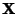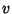## Minimax Theorem

The fundamental theorem of Game Theory which states that every Finite, Zero-Sum, two-person Game has optimal Mixed Strategies. It was proved by John von Neumannin 1928.

Formally, letandbe Mixed Strategies for players A and B. Letbe the Payoff Matrix. Thenwhereis called the Value of the Game andandare called the solutions. It also turns out that if there is more than one optimal Mixed Strategy, there are infinitely many.